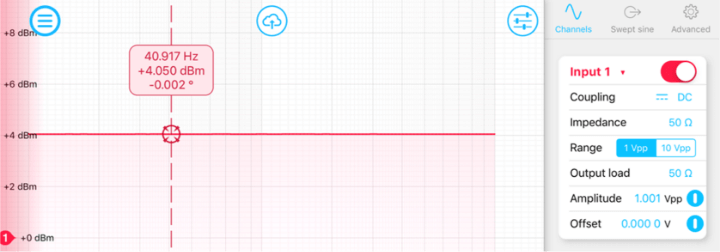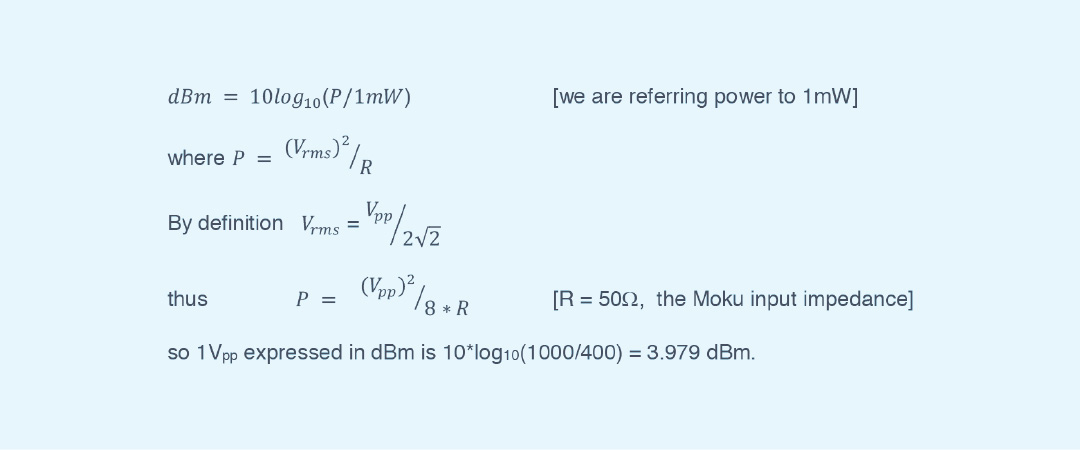# How is the magnitude in dBm calculated in Moku:Lab’s Frequency Response Analyzer?

The Frequency Response Analyzer (FRA) provides response plots of magnitude and phase. While the phase is expressed in degrees, the magnitude is expressed in terms of dBm power. This is a log scale of power expressed in dB relative to 1 milliWatt.

How is this magnitude in dBm calculated in Moku:Lab’s FRA?

Let us take a simple example. We will set the swept sine wave output of the FRA to 1 Vpp, configure 50 Ω input and output impedance, and, for this example, a sweep from 10Hz to 1kHz. Then with a coax cable connecting output 1 of the Moku:Lab to input 1, we run a sweep of the FRA. You should see a flat amplitude response at 4.0 dBm.How is the 4.0 dBm is calculated?

It comes from this formula (valid for sinusoidal signals):